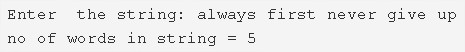# C Program Count Number Of Words In A String | 4 Ways

C program to count the total number of words in a string – In this article, we will detail in on the several means to count the total number of words in a string in C programming.

Suitable examples and sample programs have also been added so that you can understand the whole thing very clearly. The compiler has also been added with which you can execute it yourself.

The means used in this piece are as follows:

• Using Standard Method
• Using Function
• Using Recursion
• Using Pointers and While Loop

A string is nothing but an array of characters. The value of a string is determined by the terminating character. Its value is considered to be 0.As given in the image above, firstly, you need to enter a string.

The string specified here is as follows:

“always first never give up”

As you can see, there are 5 words in the given string.

It can be found out by basic reading itself.

Hence, doing the same in C programming is as follows:

## Using Standard Method

1. Read the entered string and initialize to s using gets(s).

2) We are finding the words count based on white spaces present in the given string. The ASCII value of white space is 32.

3) for loop iterates through the string with the structure for(i=0;s[i];i++),

If  ASCII value of any character of a string is equal to ASCII value of white space i.e 32, then increase the word count.

4) After all iterations of for loop increase the word count, if i>0.

5) Print the number of words present in the string.

Output:

### Using Function

1. The main() function calls the stringwordcount(char *s) function, passing the string as an argument to the function.

2) The function stringwordcount() function compares each character’s ASCII value with white space ASCII value 32.If any character is equal to white space, then it increases the word count.

3) After all iterations of for loop, if i>0 then increase the word count by 1.

4) The function returns the word count to main() function. The main() function prints the number of words present in the given string.

Output:

#### Using Recursion

1. The main() calls the function stringwordcount(char *s).

2) The function counts the number of words as

a) If the s[i] is null, then it increases the word count by 1 if i>0 and returns the word count value to the main() function.

b) If s[i] is not null, then compare ASCII value of s[i] with 32 which is the ASCII value of white space. If s[i] is equal to white space then increase the word count and call the function stringwordcount().

The function calls itself recursively until s[i] becomes to null.

Output:

##### Using Pointers And While Loop
1. The pointer variable p points the string s.

2) The while loop iterates until the character at the pointer variable become null.

a) If the ASCII value of the character at the pointer variable p is equal to white space ASCII value. Then increase the word count.

3) Increase the word count by 1 if the length of the string is greater than zero.

4) Print the number of words present in the string.

Output:

x

## C Program To Print Number Of Days In A Month | Java Tutoring

C program to input the month number and print the number of days in that ...